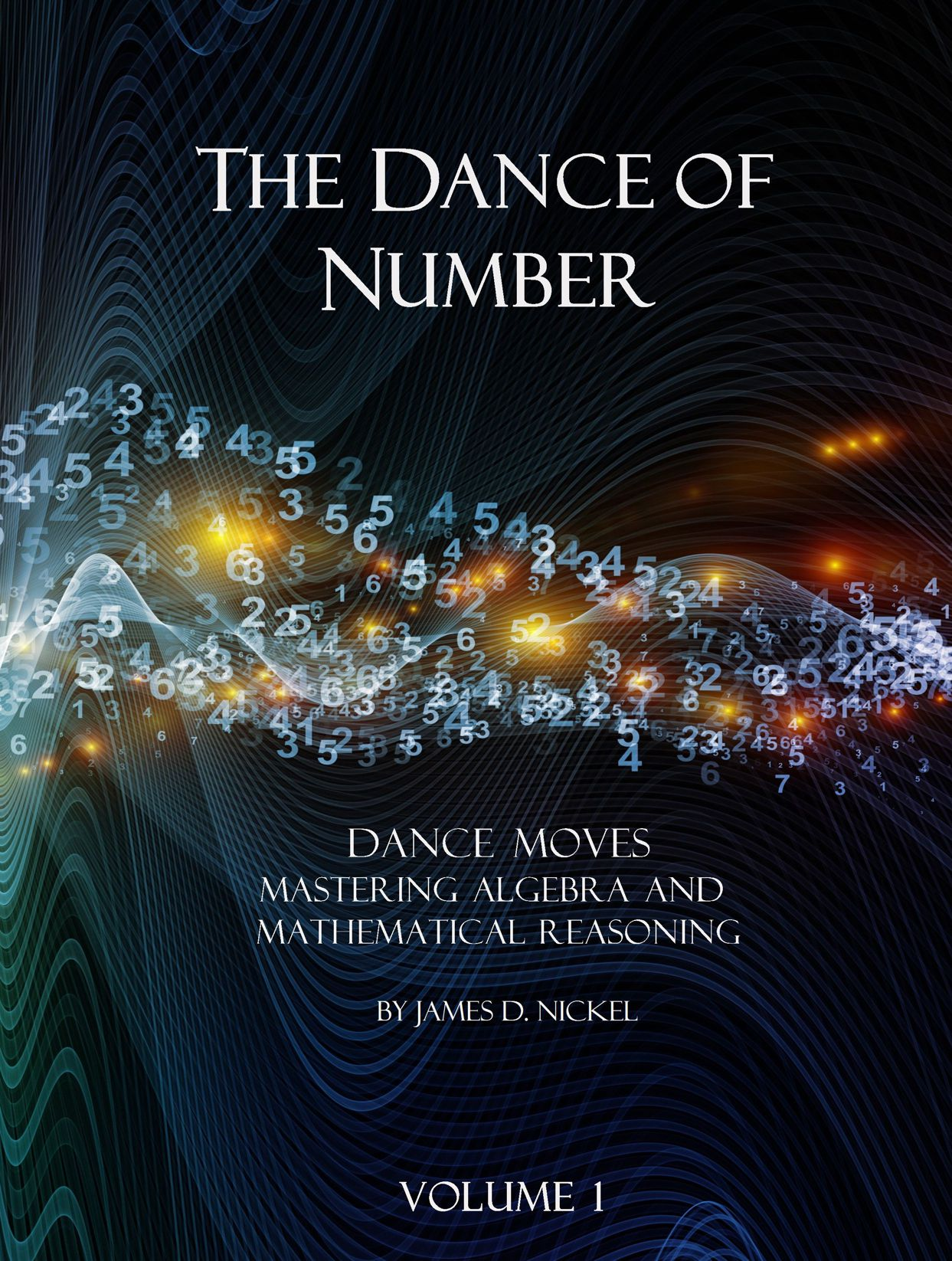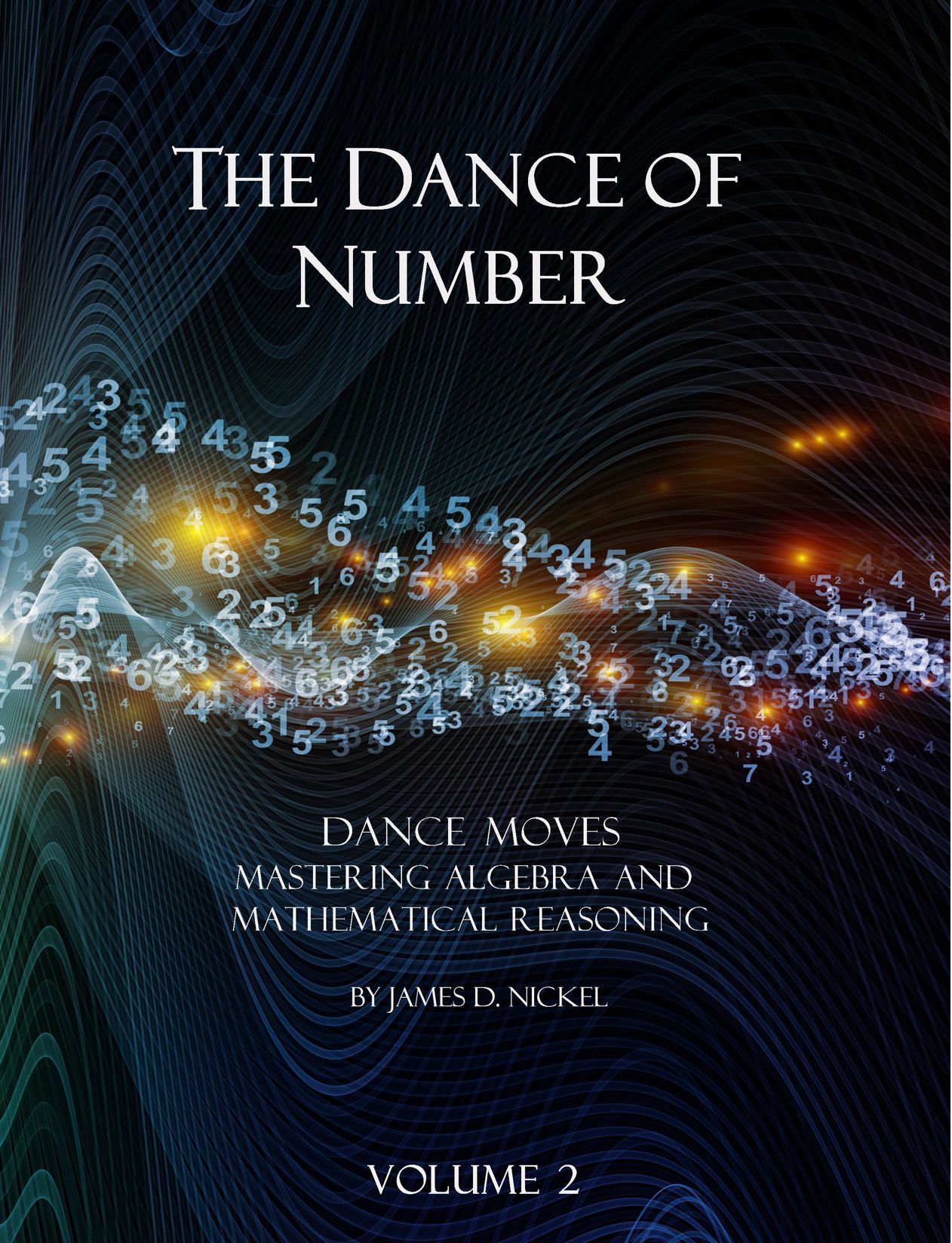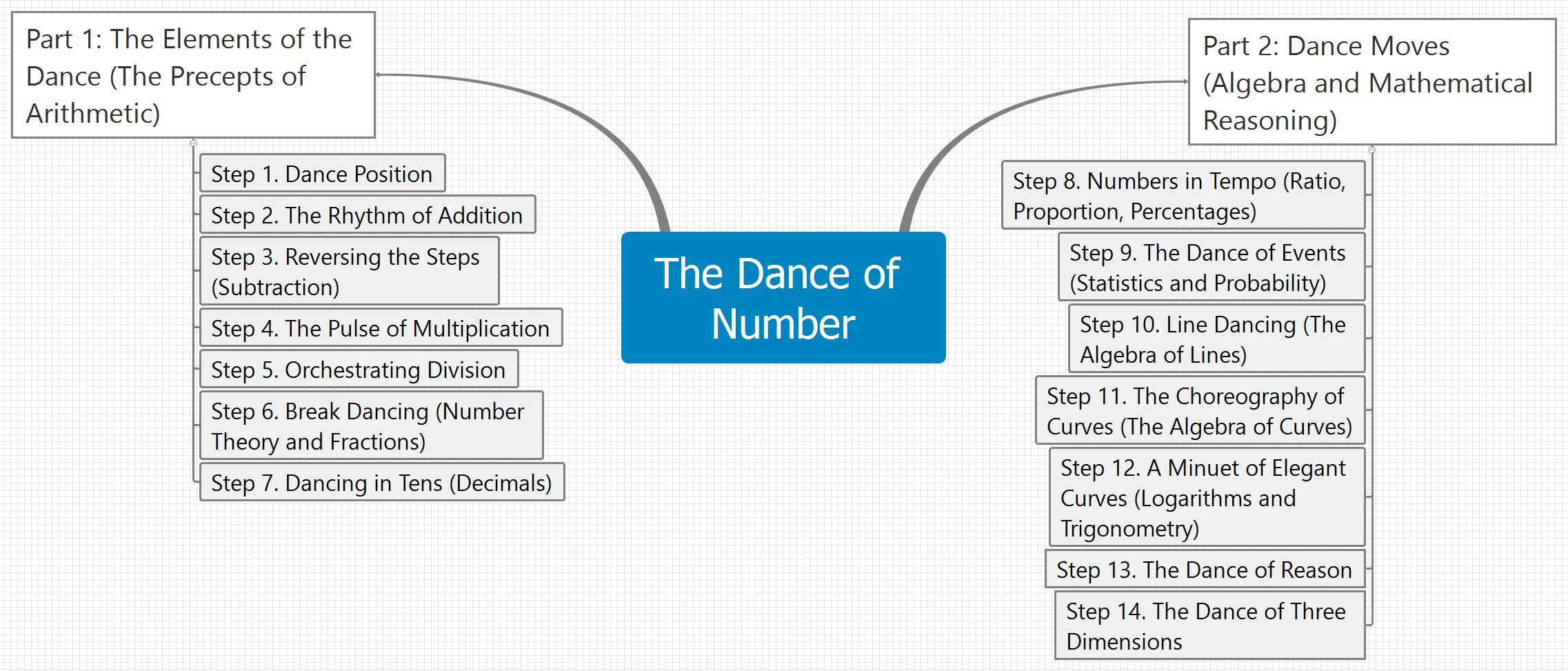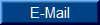## The Dance of Number Curriculum: Part 2, Steps 8-14The Dance of Number, Part 2: Suggested two-year schedule for Steps 8-14 in pdf format (*.pdf).

This Suggested two-year schedule/observations for Steps 8-14 is without indicators for quizzes/exams. The document will be updated when those assessments are ready in the spring of 2021.

Sample lesson, Lesson 9.9

Order Volume 1 text, Steps 8-11 (574 pages)

Order Solutions manual for Volume 1, Steps 8-11/Instructor's Guide (334 pages)

Sample lesson, Lesson 13.19

Order Volume 2 text, Steps 12-14 (588 pages)

Order Solutions manual for Volume 2, Steps 12-14/Instructor's Guide (334 pages)

Order Assessments (Quizzes and Exams), Steps 8-14: Forthcoming, Winter 2022

Order Computation Notebook

Software:

Graphmatica by KSoft

Ultra Fractal

Desmos

Great Stella Polyhedron Exploration

Graphing Calculator 3D

Resources:

Lesson 8.2: Algebraic History

Lesson 8.3: Classes of Levers

Lesson 8.4: Circular Measures

Lesson 8.6: Planetary Measures

Lesson 8.11: 8 by 8 Square to 5 by 13 Rectangle

Lesson 8.15: Percents, Decimals, Fractions

Lesson 8.16: Amount of Compound Interest

Lesson 9.4: Pascal's Triangle

Lesson 9.8: Pi to 2050 decimal places

Lesson 9.9: Harmonic Triangle

Lesson 10.2: Function Notation

Lesson 10.5: The Dance between Functions and their Inverse

Lesson 10.8: Absolute Value Theorem

Lesson 11.2: Eight by Eight Grid

Lesson 11.4: Direct and Indirect Problems

Lesson 11.4: Solving Word Problems

Lesson 11.6: Powers of 2 and Powers of 10 (Computer Technology)

Lesson 11.7: Squares and Square Roots

Lesson 11.13: Composition Functions

Lesson 12.2: Online Slide Rule

Lesson 12.5: Common Log Table

Lesson 12.5: The Dance between the Laws of Exponents and the Laws of Logs

Lesson 12.6: The Six Trig Ratios

Lesson 12.6: Important Trig Ratios

Lesson 12.6: The Geography of a Circle

Lesson 12.6: Trigonometry Resources from Stokes Publishing

Lesson 12.7: Sign changes for sin, cos, and tan

Lesson 12.7: Trigonometric Ratios for Quadrant I

Lesson 12.8: Electromagnetic Spectrum

Lesson 12.9: Common Angles in the Unit Circle

Lesson 12.9: Reference Angles

Lesson 12.10: Protractor

Lesson 12.11: Numbers - Natural to Complex

Lesson 12.12: Fractals

Lesson 12.12: The Koch Snowflake

Lesson 12.12: Fibonacci Numbers and the Mandelbrot Set

Lesson 13.1: The Operations of Arithmetic, their Inverses, and Evaluation/Solution Strategies

Lesson 13.2: Euclid's Axioms and Postulates

Lesson 13.2: The Beauty and Power of Mathematics

Lesson 13.5: Da Vinci's Geometric Proof of the Pythagorean Theorem

Lesson 13.8: General Forms of the Conic Sections

Lesson 13.8: Physical Properties of the Conic Sections

Lesson 13.9: Logic Gates and the Computer World

Lesson 13.10: Classical and Modern Physics

Lesson 13.15: Summary of Series Formulas

Lesson 13.16: Proof of the Binomial Theorem by Mathematical Induction (Ancilliary to the lesson for challenge work)

Lesson 13.16: Proof of the Associative Law of Addition by Mathematical Induction (Ancilliary to the lesson for challenge work)

Lesson 13.16: Polar Cooordinate Grid

Lesson 14.1: Net for Volume of a Cube

Lesson 14.1: Geometry Formulas

Lesson 14.3: Rational Functions and the Dance of their Asymptotes

Lesson 14.5: Cut and Assemble 3-D Shapes

Lesson 14.5: The Seven Crystal Systems

Lesson 14.5: Platonic Solids (Only Five)

Lesson 14.8: Mathematical Landscape

Steps 8-14: Algebra - Guide to Pronunciation

Steps 8-14: Algebraic Notation - Common Mistakes

Steps 12-14: Trig Memory Aid 3 by 5 Cards

Part 1

ERRATA (27 April 2020)

For reporting of typos, or other communication, contact the author: